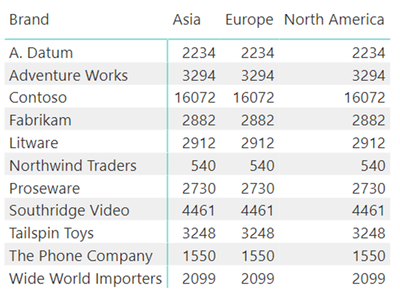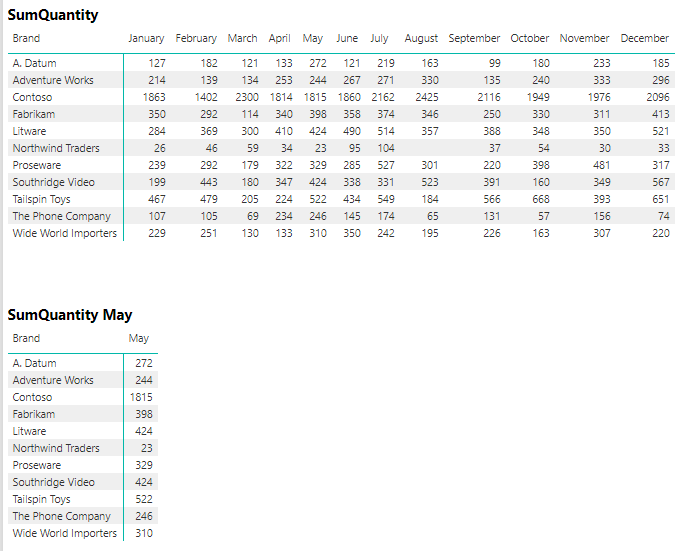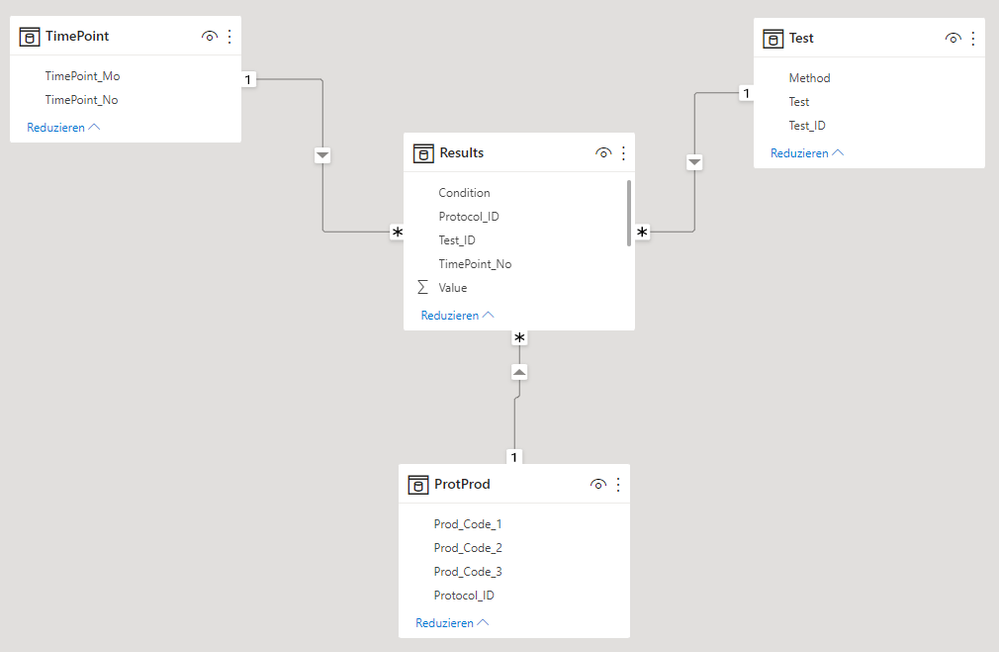cancel
Showing results for
Did you mean:Regular Visitor

## Basic CALCULATE question OR a strange phenomenon

Hello,

I have a question regarding the following simple Measure that does not give the result that I would expect. I would like to know if it is my lack of PBID / DAX understanding (I am new to it) or if there might be something wrong with my data model, PBID settings, etc.

``````Quantity A =
CALCULATE (
SUM( fctTable1[Quantity] ),
dimTable1[Column1] = "A"
)``````

I would have expected a Matrix-Visual such as the following (i.e. CALCULATE overwrites the existing filter of dimTable1[Column1] with the new filter for „A“:

(dimTable2[Column1] as rows, dimTable1[Column1] as columns)

 dimTable1[Column1] dimTable2[Column1] A B C ab 1 1 1 cd 4 4 4 ef 7 7 7

Edit: Actually this was also my intention!

However, what I got was the following Matrix-Visual (i.e. only the „A“ column was visible):

 dimTable1[Column1] dimTable2[Column1] A ab 1 cd 4 ef 7

To verify this result (in case I misunderstood the concept of CALCULATE of replacing a previously existing filter on columns contained in the filter argument) I tried the Measure in a sample file (using the contoso model) from a text book about DAX:

``````Quantity Europe =
CALCULATE (
SUM( Sales[Quantity] ),
Store[Continent] = "Europe"
)``````

Surprisingly this time the result was as expected (i.e. all columns were present in the Matrix-Visual, but showing the Quantity for Europe). This was also my original purpose.So, the same Measure leads to 2 different visuals. I’m definitely overlooking something, but what?

Has anyone a hint?

thanx

1 ACCEPTED SOLUTIONSuper User
7 REPLIES 7Super UserRegular Visitor

Thanx a lot.

I just checked this forum for sort-by-column issues, but not the web.

I definitely don’t understand the code of the queries obviously working in the background in Power Bi or Power Pivot, but I will remember to include the sort-by column in future DAX code.

In the current example it worked:Regular Visitor

Meanwhile I have found the reason for this behaviour, but I have no explanation.

The result I get, depends on if the column which is part of the CALCULATE filter argument is being sorted by another column or not.

As an example, I have used a Contoso sample file.

The measures:

``````SumQuantity =
SUM( Sales[Quantity] )

SumQuantity May =
CALCULATE(
SUM( Sales[Quantity] ),
'Date'[Month] = "May"
)``````

The visuals without sorting the 'Date'[Month] column:The visuals with sorting of the 'Date'[Month] column by Month Number:An additional Visual combining both measures in a single measure:Is there anyone out there who can explain this behaviour?

Is this normal?

Should columns not be sorted by other columns?Super UserRegular Visitor

I couldn't figure out how to attach a pbix file, so here is the basic info:

Model:Measures:

``````SumValue =
SUM( Results[Value] )

SumValue Start =
CALCULATE(
SUM( Results[Value] ),
TimePoint[TimePoint_Mo] = "Start"
)

SumValue Start FILTER =
CALCULATE(
SUM( Results[Value] ),
FILTER(
TimePoint,
TimePoint[TimePoint_Mo] = "Start"
)
)

SumValue Start FILTER wALL =
CALCULATE(
SUM( Results[Value] ),
FILTER(
ALL( TimePoint[TimePoint_Mo] ),
TimePoint[TimePoint_Mo] = "Start"
)
)``````

VisualsMeanwhile, my first question is, what should be the result of following measure using a matrix-visual?

``````SumValue Start =
CALCULATE(
SUM( Results[Value] ),
TimePoint[TimePoint_Mo] = "Start"
)``````

What data should be displayed?Super User

@NoIdea . Based on what I got, Try like

Quantity Europe =
CALCULATE (
SUM( Sales[Quantity] ),
Filter(Stores, Store[Continent] = "Europe")
)

http://dataap.org/blog/2019/04/22/difference-between-calculate-with-and-without-filter-expression/

!! Microsoft Fabric !!
Microsoft Power BI Learning Resources, 2023 !!
Learn Power BI - Full Course with Dec-2022, with Window, Index, Offset, 100+ Topics !!
Did I answer your question? Mark my post as a solution! Appreciate your Kudos !! Proud to be a Super User! !!Regular Visitor

However, I should have explained my original intention a bit more.

My intention was that the mentioned Measure diplays the same value for all fields in the Matrix-Visual. The problem was, that it did not do it, as I was expecting.

I think I understand the difference to your measure (i.e. using FILTER, because CALCULATE evaluates its filter arguments in the original evaluation context).

However, when I understand correctly my measure could be written with FILTER as well:

``````Quantity Europe =
CALCULATE (
SUM( Sales[Quantity] ),
Store[Continent] = "Europe"
)

Is the same as

Quantity Europe FILTER =
CALCULATE (
SUM( Sales[Quantity] ),
FILTER(
ALL( Store[Continent] ),
Store[Continent] = "Europe"
)
)``````

But in my data model (not the contose sample file) it looks like as if the measure

``````Quantity Europe =
CALCULATE (
SUM( Sales[Quantity] ),
Store[Continent] = "Europe"
)``````

behaves like this measure

``````Quantity Europe FILTER woALL =
CALCULATE (
SUM( Sales[Quantity] ),
FILTER(
Store,
Store[Continent] = "Europe"
)
)``````

which produces this result, that I do not want: